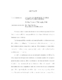## A Class of Stable, Efficient Navier-Stokes Solvers##### Files
umi-umd-3470.pdf(2.22 MB)
We study a class of numerical schemes for Navier-Stokes equations (NSE) or Stokes equations (SE) for incompressible fluids in a bounded domain with given boundary value of velocity. The incompressibility constraint and non-slip boundary condition have made this problem very challenging. Their treatment by finite element method leads to the well-known inf-sup compatibility condition. Their treatment by finite difference method leads to the very popular projection method, which suffers from low resolution near the boundary. In [LLP], the authors propose an unconstrained formulation of NSE or SE, which replace the divergence-free constraint by a pressure equation with an appropriate boundary condition. All of the schemes in this thesis are based on this new formulation. In contrast to traditional methods, these schemes do not need to fulfill the traditional inf-sup compatibility condition between velocity space and pressure space. More importantly, they can achieve high-order accuracy very easily and are very efficient due to the decoupling of the update of velocity and pressure. They can even be proved to be unconditionally stable. There are two ways to analyze the schemes that we propose. The first is based upon the sharp estimate of the pressure in [LLP]. The second relies on a nice identity. Using the pressure estimates, we propose and study a $C^1$ finite element (FE) scheme for the steady-state SE as well as for the time-dependent NSE. For steady-state SE, we can either use an iterative scheme or solve velocity and pressure together. Using the nice identity, we prove that the semi-discrete iterative scheme for the steady-state SE converges ("semi-discrete" means that the spatial variable are kept continuous). This identity will also be crucial for our proofs of the stability and error estimates of the time-dependent $C^0$ FE schemes. Associated numerical computations demonstrate stability and accuracy of these schemes. We also present the numerical results of yet another $C^0$ FE scheme ([JL]) for the time-dependent NSE for which the theory of the fully discrete case is yet lacking.